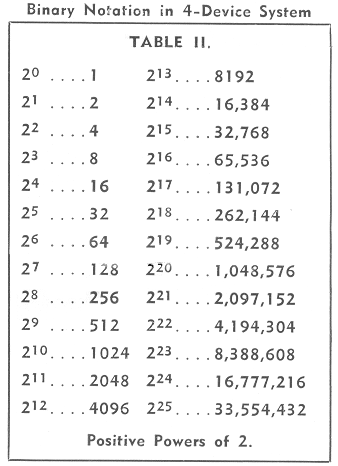AEROTEK ELEMENTARY BINARY ARITHMETIC
 [From The Aerovox Research Worker, December 1954. Typographical errors in images have been manually corrected.]ELEMENTARY BINARY ARITHMETIC By the Engineering Department, Aerovox Corporation With the growing presence of digital electronic computers among us, more and more radio technicians are beginning to hear obliquely about the binary number system and wonder why they have learned nothing about it before. There is good excuse for the perplexity, since surprisingly little has appeared on the subject in the books and magazines customarily read by radio men. Many technicians who have prided themselves on being reasonably well grounded have thumbed through mathematics textbooks, old and new, and found no reference whatever to the binary system. A glance into the dictionary reveals the word binary to mean "characterized by two things or parts." From this, we may infer, correctly, that binary arithmetic is in some way associated with the figure 2. Indeed, the binary system uses only two digits. Now, let us see how this differs from the method of counting we have employed most of our lives. Our old standby is the decimal system. Its base is 10 and its digits are 0, 1, 2, 3, 4, 5, 6, 7, 8, and 9. This is very handy because we have ten fingers on which to count. In our civilization, we have gotten along famously with the base 10. It is possible to express any number by the proper combination of the digits 0 to 9. However, when we attempt to set up some forms of electrical counting equipment in strict accordance with the decimal system, we find ourselves in need of a multitude of components. Here, the binary method comes to our rescue. It is a base 2 system and requires only two digits: 0 and 1. In the binary system, all numbers can be expressed by combinations of zeros and ones. Just why should this be handier than than the decimal system? Simply because it is an easy matter to express the binary digits themselves with a simple electrical device which is either ON (1) or OFF (0). Thus, an open switch or relay denotes zero, while a closed switch signifies 1. The same is true of a tube conducting or cut off, a crystal diode conducting or blocking, a neon lamp ignited or extinguished, etc., etc. A voltage or current likewise can denote 1 when high or positive, and zero when low, negative, or off. The binary system operates with fewer and simpler components. Although a certain piece of equipment, such as a counter, might operate by the binary method, it still can be made to give indications (such as total count) in the easily-recognized decimal notation. In explaining the elements of binary arithmetic in this article, frequent comparisons will be made with the decimal system for the sake of clarity or proof.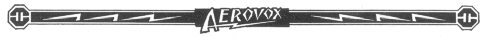Rudiments of the System Suppose that you have four separate on-off components (switches, tubes, etc.), each of which is assumed to indicate zero when OFF and 1 when ON. Table I shows how the two states of these same four devices can be employed to express various decimal numbers. In order better to understand this table, let us consider the basic rules of binary addition which may be stated as follows: 0 + 0 = 0, 0 + 1 = 1, 1 + 0 = 1, and 1 + 1 = 10. This last sum means simply that every time 1 is added to 1, we write down zero and carry the 1 to the next column to the left. An illustration will serve to clarify binary addition. For serve to clarify binary addition. For example, from Table I add 0101 (binary 5) and 0011 (binary 3):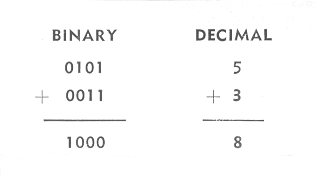First, the two 1's in the right-hand column are added. This equals 10, so we write 0 amd carry 1 to the next column to the left. This 1 must be added to the 1 already in that column. Again, this equals 10, so we write 0 and carry 1 to the next column to the left. Adding this carried 1 to the 1 already in that column gives another 10, so we write another zero and carry 1 to the left-most column. Now, this 1 is added to the zero in that column, giving 1 which is written. The answer is 1000, which by reference to Table I is found to be binary 8. A careful examination of Table I now reveals that each higher binary number is obtained by adding binary 1 (0001) to the preceding number. Try this out by starting with 1010 (binary 10) and successively adding 0001 (binary 1). You will obtain 1011 from 11, 1100 for 12, 1101 for 13, 1110 for 14, and 1111 for 15. If you make another addition, you will obtain 10000 (binary 16), which requires five on-off devices for its expression - and we agreed at the beginning that we have only four. So binary 15 is as high as a 4-device system will count. However, the economy and efficiency of the system is realized when it is considered that only four elements are needed to display from 0 to 15 events. Any number may be expressed in the binary system by choice and position of the two digits repeated as coefficients of powers of 2, just as any number can be expressed in the decimal system by choice and position of the ten digits of that system as coefficients of powers of 10. For example: The decimal number 2548 means 2 × 103 + 5 × 102 + 4 × 101 + 8 × 100. Similarly, the binary number 011010 means 0 × 25 + 1 × 24 + 1 × 23 + 0 × 22 + 1 × 21 + 0 × 20. Table II lists the powers of 2 up to 225, and you can obtain from this Table the decimal numbers corresponding to the powers of 2 given in the preceding example. Adding these discloses that 011010 equals 26: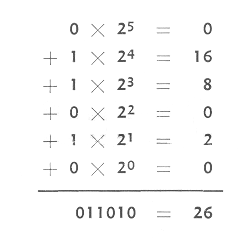You can prove this sum by returning to Table I and adding binary 1 (0001) successively to binary 10 until you reach 011010 which you will find equal to 26. A binary point is used in binary notation just as a decimal point is used in decimal notation. An example is 100101.01 with six digits on the left and two on the right of the binary point, although the digits might increase in number without limit on both sides of the point. We have seen already that the digits on the left of the point are coefficients of increasing positive powers of 2 with 20 adjacent to the binary point. The digits on the right are coefficients of increasing negative powers of 2 with 2-1 adjacent to the point. The example just given (100101.01) becomes: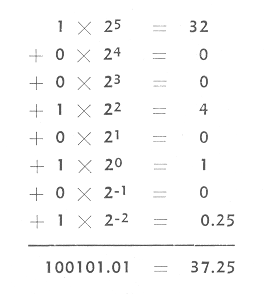Binary Addition The addition of positive numbers in the binary system already has been explained in the preceding paragraphs. While the addition of two numbers has been given in each illustration, the system is by no means restricted to 2-number groups. Any series of binary numbers can be summed. The only remaining case is the addition of a positive and a negative number. Consider, for example, the addition of 0101 and -0010: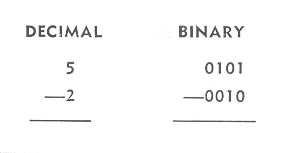The technique is to change the sign of the negative number, then complement this number, and add the result to the positive number. To complement the number, change each of its 1's to 0's and each of its 0's to 1's and add 1. Thus, -0010 becomes: 1101 + 1 = 1110. Now if we add: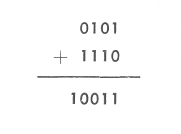Here, the left-most digit in the answer is discarded. If it is 1, the sign of the answer is positive, as in the above case. The answer thus is + 0011, or binary 3 which satisfies the cibdutuib if 5 - 2 = 3. If the left-most digit is zero, the sign of the answer is negative and the result must be recomplemented (the same process as the original complementing) to give the correct answer. This always happens when a negative number is added to a smaller positive number. Thus: Add -1000 (binary 8) to 0011 (binary 2):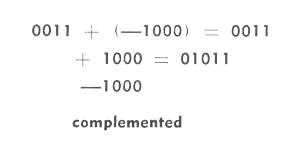Dropping the left-most 0 in the answer (which merely indicates the negative sign), and recomplementing changes 1011 to -0101 (binary 5), which is the correct answer, -8 + 3 = -5.Binary Subtraction Subtraction is the same as the addition of positive and negative binary numbers, as just described. For example: Subtract 0100 (binary 4) from 1010 (binary 10). 1010 - 0100 becomes 1010 + 1100 when the negative number (subtrahend) is complemented and its sign changed. This equals 10110. The left-most digit, being a 1, indicates that the sign of the answer is positive and is discarded, making the answer +0110 (binary 6) which is correct.Binary Multiplication Binary multiplication is carried out in very much the same manner as decimal multiplication, obtaining partial products in the conventional manner, but adding the latter in binary fashion. In binary multiplication, 0 × 0 = 0, 0 × 1 = 0, 1 × 0 = 0, and 1 × 1 = 1. As an example, multiply 0101 (binary 5) by 0010 (binary 2):Binary Division Binary division is carried out in a manner similar to decimal division, as the following example will show: Divide 1001 (binary 9) by 0100 (binary 4):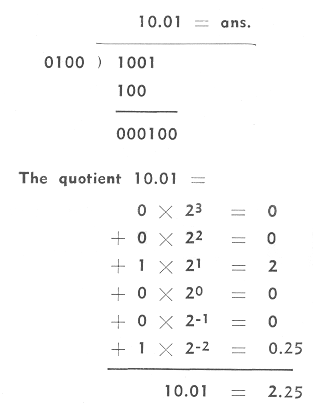Conclusion After studying the rudiments of binary arithmetic presented here, the reader should be able, by setting up for himself a number of practice examples for drill, to acquire considerable proficiency in manipulating this invaluable new tool. A good working knowledge of the binary system is essential to comprehending the operation of digital electronic computers and of other instruments, such as counters, which utilize the digital techniques. Such devices will be described in future issues of the Research Worker.